Skip to main content

# Main Classification Algorithms - Part 1Classification is a method used in Supervised Learning, which aims at outputting a discrete variable, such as a class or a label. Hence, the model is trained in such a way to render a discrete output (y) for some input data (x).

In the previous article, we had focused on the classification evaluation metrics and classification measures, which are essential to evaluate the performance of the model at hand.

Below, we will take a look at some of the main classification algorithms such as the K-nearest neighbors (KNN), Decision trees and Random Forests. This article has a second part, focusing on Logistic Regression and Naïve Bayes algorithms. So keep an eye out for this upcoming post.

### Main Classification algorithms

1.a. K-nearest neighbors (KNN)

The K-nearest neighbors (KNN) algorithm as a type of supervised machine learning algorithm that can be used both for regression and classification, but is mostly used in the latter. KNN algorithms trains on a labeled dataset and then attempts to predict the correct class for the test data, by calculating the distance between the test input and the training points. In other words, the KNN algorithm stores the training points and then classifies a new data point based on the similarity with the training points.

Below is an example of a KNN output with three neighbors i.e. three classes. The goal is to identify to which class the point X belongs to.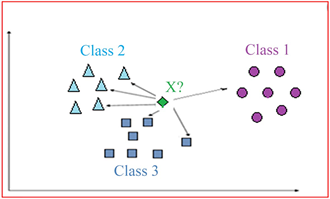The algorithm for the KNN models are as follows:

1. Select the number K of neighbors

2. Calculate the Euclidean distance of K number of neighbors

3. Take the K nearest neighbors as per the calculated Euclidean distance

4. Among these K neighbors, count the number of data points in each category

5. Assign the new data point to that category for which the number of neighbors is maximal

6. The model is completed

The Euclidean distance can be calculated as follows:       ​​​​​​​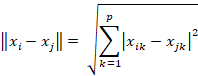where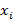and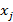are vectors with p components. The Euclidean distance is a special case of the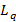distance, with q = 2.​​​​​​​​​​​​​​

1. b. Decision Trees

A decision tree is a predictive modelling tool that can be used for both regression and classification models, but is most often used for the latter. In the case of classification decision trees, the decision variable is categorical, while in regression decision trees, the decision variable is continuous. Decision trees are probably the most understandable machine learning algorithms and can be easily interpreted. These trees can be constructed by an algorithmic approach that will split the dataset into different ways based on certain conditions. In such a tree, each node represents a predictor variable or feature, the link between each node is called a branch and represents a decision, while each leaf node represents an outcome or response variable.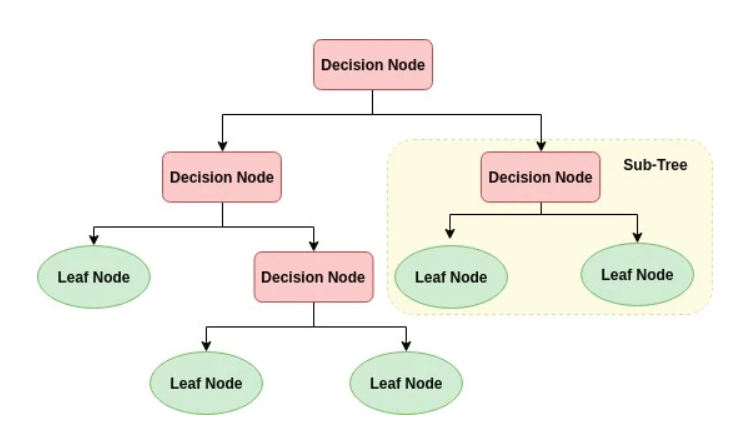###### Components of a decision tree

The making of a decision tree algorithm involves the following steps:

1. Select the predictor variable that best classify the dataset into the desired classes and assign this feature to be the root node i.e. the first node at the top of the tree.

2. Travel down from the root node, while making decisions at each internal node, such that each node best classifies the data.

3. Go back up to the first step and repeat the process until we can assign a class to the input data.

To evaluate binary splits in decision tree algorithms, we use the Gini index as a cost function. This function works with categorical target variables and can be computed as follows: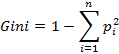where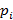is the probability of an element being classified to a particular class.

While building a decision tree, we would aim to choose features with the least Gini index as the root node.

Another splitting measure that can be used for decision tree algorithms in the information gain. Indeed, it is used to determine which feature gives us the maximum information about a class or final outcome. It uses the concept of entropy, which measures the impurity or uncertainty in the data and is used to determine how the decision tree can split the data. Below are the formulas for entropy and information gain: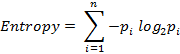​​​​​​​​​​​​​​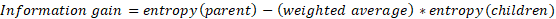1.c. Random Forest

We have just understood the functioning of Decision Tree algorithms. Based on this, we can now examine Random Forest algorithms. Indeed, Random Forest is a supervised learning algorithm, which can be used for both classification and regression problems, but it remains mainly used in classification. This kind of algorithm creates a set of randomized decision trees on data samples, identifies the best predictions from each of them and then selects the best solution through voting. Thus, it involves multiple trees producing different values, which reduces the overfitting of the algorithm by averaging the results.

Random forest algorithms can be summarized with the following steps:

1. Select random samples from a given dataset

2. The algorithm then constructs a decision tree for every sample and gets the prediction results from every tree

3. Voting is performed for every predicted result obtained

4. Select the most voted prediction result as the final prediction result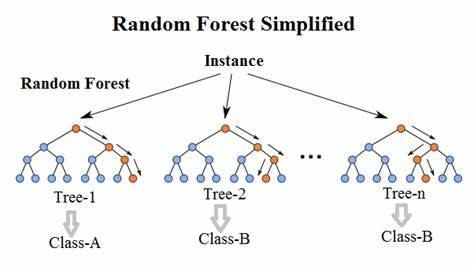###### Example: Random forest with three decision trees

Random forest algorithms have been shown to be of high efficacy in fraud detection, compared to other models. Indeed, in a study conducted by Dal Pozzolo et al (2014), authors looked at credit card fraud detection and compared multiple algorithms. The results showed that a Random Forest based model outperforms a support vector machine and even a neural network in terms of AP, AUC and Precision Rank metrics.

###​​​​​​​What’s next

Hopefully, this article will have given you a good understanding of the first three classification algorithms used and some of their possible applications.

The following article constitutes a part 2 to this article and will be focusing on Logistic Regression and Naïve Bayes algorithms : two classification methods.

### Ref

Dal Pozzolo, A., Caelen, O., Le Borgne, Y. A., Waterschoot, S., & Bontempi, G. (2014). Learned lessons in credit card fraud detection from a practitioner perspective. Expert Systems with Applications, 41(10), 4915 4928. https://doi.org/10.1016/j.eswa.2014.02.026